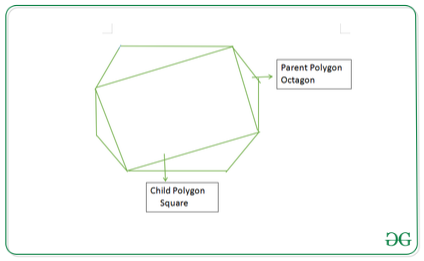Related Articles

# Polygon with maximum sides that can be inscribed in an N-sided regular polygon

• Difficulty Level : Easy
• Last Updated : 24 Mar, 2021

Given a regular polygon of N sides, the task is to find the maximum sided polygon that can be inscribed inside the given polygon by joining non-adjacent vertices. Print -1, if no such polygon exist.

Examples:

Input: N = 8
Output: 4
Explanation:
At most a 4 sided polygon can be inscribed inside the given 8-sided polygon as shown below:Input: N = 3
Output: -1

Approach: The idea is to observe the fact that a regular polygon can be inscribed inside another regular polygon of N sides if N is even. Follow the below steps to solve the problem:

1. If N is even, then the inscribed polygon with the maximum sides can be formed by joining the non-adjacent vertices. Therefore, print N/2 as the required answer.
2. Otherwise, print -1 as no regular polygon can be inscribed inside an odd-sided polygon.

Below is the implementation of the above approach:

## C++

 `// C++ program for the above approach` `#include ``using` `namespace` `std;` `// Function to find the maximum``// sided polygon that can be inscribed``int` `MaximumSides(``int` `n)``{``    ``// Base Case``    ``if` `(n < 4)``        ``return` `-1;` `    ``// Return n/2 if n is even``    ``// Otherwise, return -1``    ``return` `n % 2 == 0 ? n / 2 : -1;``}` `// Driver Code``int` `main()``{``    ``// Given N``    ``int` `N = 8;` `    ``// Function Call``    ``cout << MaximumSides(N);` `    ``return` `0;``}`

## Java

 `// Java program for the``// above approach``import` `java.util.*;``class` `GFG{` `// Function to find the``// maximum sided polygon``// that can be inscribed``static` `int` `MaximumSides(``int` `n)``{``  ``// Base Case``  ``if` `(n < ``4``)``    ``return` `-``1``;` `  ``// Return n/2 if n is``  ``// even Otherwise, return -1``  ``return` `n % ``2` `== ``0` `?``         ``n / ``2` `: -``1``;``}` `// Driver Code``public` `static` `void` `main(String[] args)``{``  ``// Given N``  ``int` `N = ``8``;` `  ``// Function Call``  ``System.out.print(MaximumSides(N));``}``}` `// This code is contributed by shikhasingrajput`

## Python3

 `# Python3 program for the above approach` `# Function to find the maximum sided``# polygon that can be inscribed``def` `MaximumSides(n):``    ` `    ``# Base Case``    ``if` `(n < ``4``):``        ``return` `-``1` `    ``# Return n/2 if n is even``    ``# Otherwise, return -1``    ``if` `n ``%` `2` `=``=` `0``:``        ``return` `n ``/``/` `2``        ` `    ``return`  `-``1` `# Driver Code``if` `__name__ ``=``=` `'__main__'``:``    ` `    ``# Given N``    ``N ``=` `8` `    ``# Function Call``    ``print``(MaximumSides(N))` `# This code is contributed by mohit kumar 29`

## C#

 `// C# program for the``// above approach``using` `System;``class` `GFG{` `// Function to find the``// maximum sided polygon``// that can be inscribed``static` `int` `MaximumSides(``int` `n)``{``  ``// Base Case``  ``if` `(n < 4)``    ``return` `-1;` `  ``// Return n/2 if n is``  ``// even Otherwise, return -1``  ``return` `n % 2 == 0 ?``         ``n / 2 : -1;``}` `// Driver Code``public` `static` `void` `Main(String[] args)``{``  ``// Given N``  ``int` `N = 8;` `  ``// Function Call``  ``Console.Write(MaximumSides(N));``}``}` `// This code is contributed by shikhasingrajput`

## Javascript

 ``

Output:

`4`

Time Complexity: O(1)
Auxiliary Space: O(1)

Attention reader! Don’t stop learning now. Get hold of all the important mathematical concepts for competitive programming with the Essential Maths for CP Course at a student-friendly price. To complete your preparation from learning a language to DS Algo and many more,  please refer Complete Interview Preparation Course.

My Personal Notes arrow_drop_up